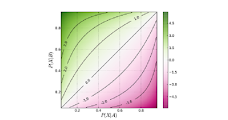## Odd's Ratio

DEFINITION:
• ODDS:The probability that an event will occur divided by the probability that the event will not occur
• Odds = Probability/(1-probability)
• RATIO:Is the number (a) of observations in a given group with a given characteristic ( such as having an MI) divided by the number (b) of observations without the given characteristic (such as not having MI). v ODDS RATIO: Is the ODDS that a patient is exposed to the risk factor divided by the ODDS that a control is exposed
Odd ratio in case control study:
Case control
Exposed a b
unexposed c d
• Odd of exposure among the cases : a/c
• Odd of exposure among the control: b/d
• Therefore Exposure odd ratio is: a/c ⁄b/d
b/d bc

• In a case control study, the most characteristic feature is odd's ratio
Odds ratio in cohort study:
Outcome YES Outcome No
Exposed a b
unexposed c d
• Odd of outcome among exposed=a/b
• Odd of outcome among unexposed=c/d
c/d

• Odds ratio can be calculated in a cohort study and in a case-control study.
• The exposure odds ratio is equal to the disease odds ratio.
• Relative risk can only be calculated in a cohort study.
• Odds ratio can be a measure of relative risk in case control study.
Odds ratio is a measure of true relative risk when:
• The outcome is relatively rare. ( prevalence is 10% or less in general population)
• The cases must be representative of the cases in the population.
• The controls must be representative of the controls in the population
Interpretation of odds ratio (OR) :
• OR of >1 indicates that the exposure is associated with an increased risk of developing the disease.
• OR of Closer the value of OR to 0 greater the protection.
• The OR will rarely equal to 1 in absence of true risk or protection
Uses of OR
• OR are appropriate measure of RR in case control studies.
• OR are commonly used in meta analysis.
• Odds ratio is the measure of the strength of the association between risk factor and outcome.
• OR are the output of logistic regression analysis.